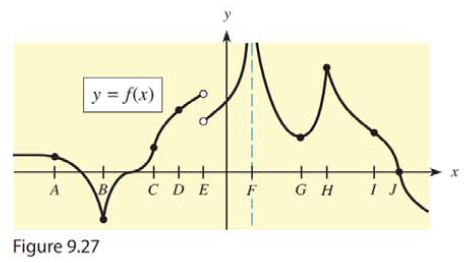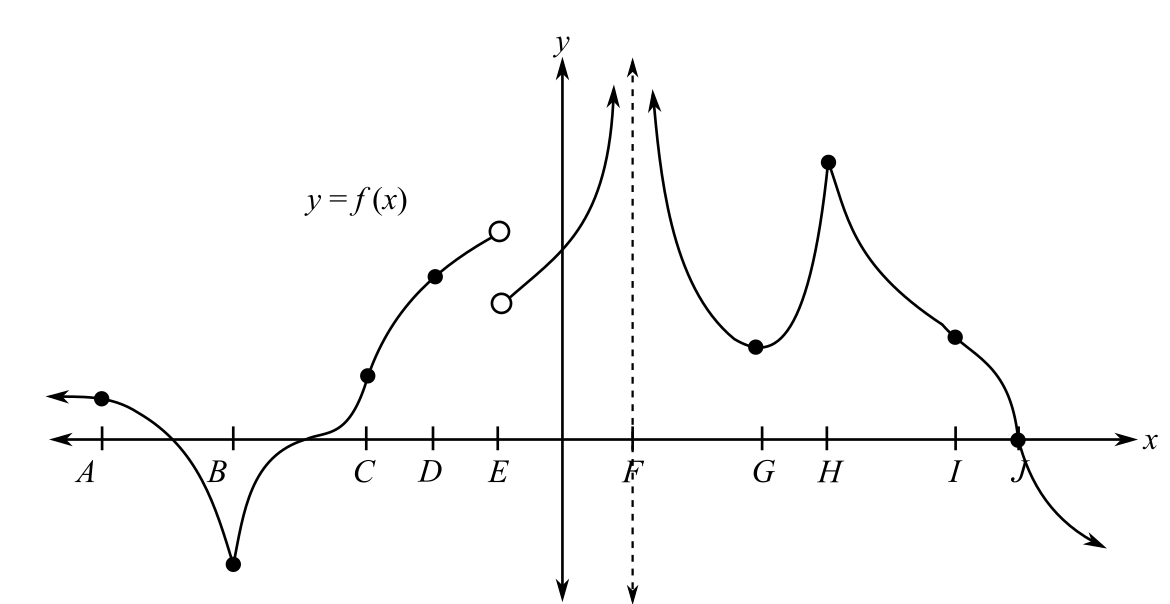Chapter 9.3, Problem 33EMathematical Applications for the ...

12th Edition
Ronald J. Harshbarger + 1 other
ISBN: 9781337625340

Solutions

Chapter
SectionMathematical Applications for the ...

12th Edition
Ronald J. Harshbarger + 1 other
ISBN: 9781337625340
Textbook Problem

Given the graph of y = f ( x ) in Figure 9.27, determine for which x-values A, B, C, D, or E the function is (a) continuous. (b) differentiable.(a)

To determine

The values of x out of A,B,C,D,E for which the function is continuous in the figure.Explanation

Given Information:

The graph is given as;

Explanation:

The graph is given as;

For a function to be continuous at a point, the left-hand limit should be equal to right hand limit and its value must be the value of the function at that point.

In case of graph, continuity of the left hand and right-hand limit is observed when the graph is continuous at the given point...

(b)

To determine

The values of x out of A,B,C,D,E for which the function is differentiable in the figure.Still sussing out bartleby?

Check out a sample textbook solution.

See a sample solution

The Solution to Your Study Problems

Bartleby provides explanations to thousands of textbook problems written by our experts, many with advanced degrees!

Get Started

Evaluate the integral. 01(1+r)3dr

Single Variable Calculus: Early Transcendentals, Volume I

Evaluate the definite integral. 01xex2dx

Single Variable Calculus: Early Transcendentals

The area of the region at the right is:

Study Guide for Stewart's Multivariable Calculus, 8th